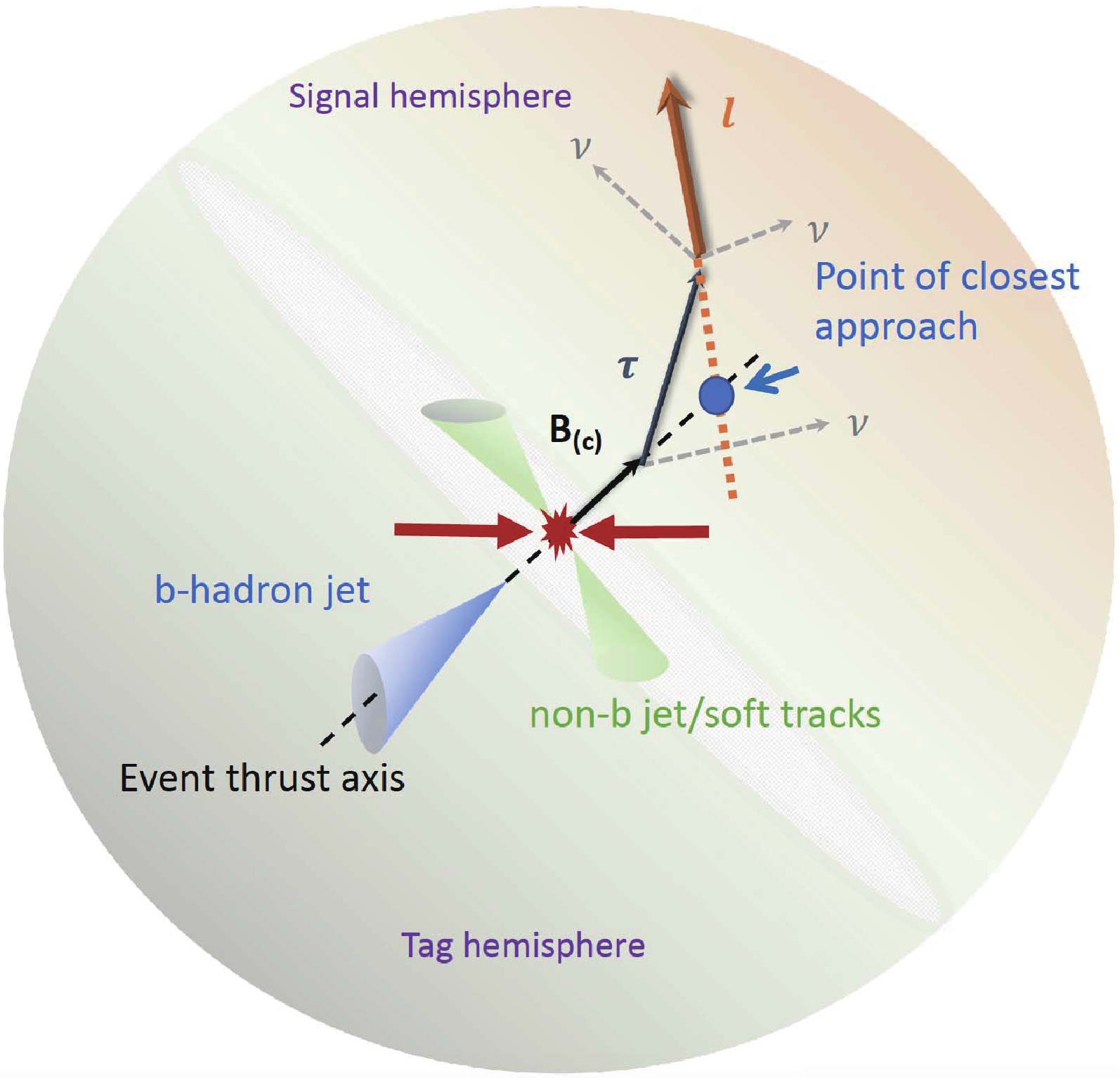# Analysis of Bc → τντ at CEPC• Precise determination of the $B_c \to \tau\nu_\tau$branching ratio provides an advantageous opportunity for understanding the electroweak structure of the Standard Model, measuring the CKM matrix element $|V_{cb}|$, and probing new physics models. In this paper, we discuss the potential of measuring the process $B_c \to \tau\nu_\tau$with $\tau$decaying leptonically at the proposed Circular Electron Positron Collider (CEPC). We conclude that during the Z pole operation, the channel signal can achieve five-$\sigma$significance with $\sim 10^9$Z decays, and the signal strength accuracies for $B_c \to \tau\nu_\tau$can reach around 1% level at the nominal CEPC Z pole statistics of one trillion Z decays, assuming the total $B_c \to \tau \nu_\tau$yield is $3.6 \times 10^6$. Our theoretical analysis indicates the accuracy could provide a strong constraint on the general effective Hamiltonian for the $b \to c\tau\nu$transition. If the total $B_c$yield can be determined to ${\cal{O}}$(1%) level of accuracy in the future, these results also imply $|V_{cb}|$could be measured up to ${\cal{O}}$(1%) level of accuracy.
••Get Citation
Taifan Zheng, Ji Xu, Lu Cao, Dan Yu, Wei Wang, Soeren Prell, Yeuk-Kwan E. Cheung and Manqi Ruan. Analysis of Bcτντ at CEPC[J]. Chinese Physics C. doi: 10.1088/1674-1137/abcf1f
Taifan Zheng, Ji Xu, Lu Cao, Dan Yu, Wei Wang, Soeren Prell, Yeuk-Kwan E. Cheung and Manqi Ruan. Analysis of Bcτντ at CEPC[J]. Chinese Physics C.Milestone
Article Metric

Article Views(292)
Cited by(0)
Policy on re-use
To reuse of Open Access content published by CPC, for content published under the terms of the Creative Commons Attribution 3.0 license (“CC CY”), the users don’t need to request permission to copy, distribute and display the final published version of the article and to create derivative works, subject to appropriate attribution.
###### 通讯作者: 陈斌, bchen63@163.com
• 1.

沈阳化工大学材料科学与工程学院 沈阳 110142

Title:
Email:

## Analysis of Bc → τντ at CEPC

• 1. School of Physics, Nanjing University, Nanjing 210023, China
• 2. INPAC, SKLPPC, MOE KLPPC, School of Physics and Astronomy, Shanghai Jiao Tong University, Shanghai 200240, China
• 3. Physikalisches Institut der Rheinischen Friedrich-Wilhelms-Universität Bonn, 53115 Bonn, Germany
• 4. Institute of High Energy Physics, Beijing 100049, China
• 5. Department of Physics and Astronomy, Iowa State University, Ames, IA, USA

Abstract: Precise determination of the $B_c \to \tau\nu_\tau$branching ratio provides an advantageous opportunity for understanding the electroweak structure of the Standard Model, measuring the CKM matrix element $|V_{cb}|$, and probing new physics models. In this paper, we discuss the potential of measuring the process $B_c \to \tau\nu_\tau$with $\tau$decaying leptonically at the proposed Circular Electron Positron Collider (CEPC). We conclude that during the Z pole operation, the channel signal can achieve five-$\sigma$significance with $\sim 10^9$Z decays, and the signal strength accuracies for $B_c \to \tau\nu_\tau$can reach around 1% level at the nominal CEPC Z pole statistics of one trillion Z decays, assuming the total $B_c \to \tau \nu_\tau$yield is $3.6 \times 10^6$. Our theoretical analysis indicates the accuracy could provide a strong constraint on the general effective Hamiltonian for the $b \to c\tau\nu$transition. If the total $B_c$yield can be determined to ${\cal{O}}$(1%) level of accuracy in the future, these results also imply $|V_{cb}|$could be measured up to ${\cal{O}}$(1%) level of accuracy.

### HTMLI.   INTRODUCTIONII.   ${{B^+_c}} {{\to \tau^+}} {{\nu_\tau}}$ IN THE SM AND IN NP MODELSIII.   DETECTOR, SOFTWARE AND SAMPLEIV.   ANALYSIS METHOD AND RESULTS
Reference (28)
PDF查看关注分享

Top

### 目录/DownLoad:  Full-Size Img  PowerPoint